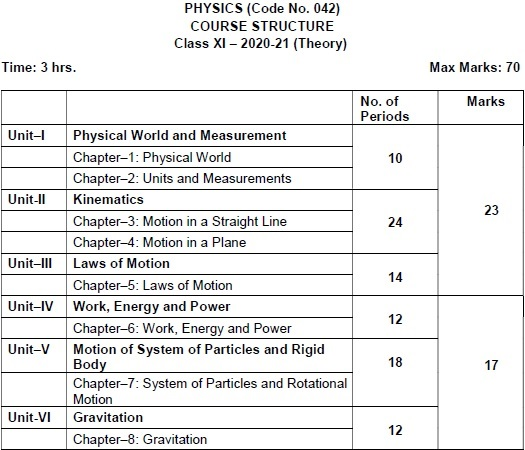Class 11 : Physics Syllabus (2020-21) For CBSE Exam | (CODE NO. 042)
Vyakhyaedu

Class 11 : Physics Syllabus (2020-21) For CBSE  Exam | (CODE NO. 042) | Vyakhyaedu

Senior Secondary stage of school education is a stage of transition from general education to discipline-based focus on curriculum. The present updated syllabus keeps in view the rigour and depth of disciplinary approach as well as the comprehension level of learners.PRACTICALS

The record, to be submitted by the students, at the time of their annual examination, has to include:
• Record of at least 12 Experiments [with 6 from each section], to be performed by the students.
• Record of at least 6 Activities [with 3 each from section A and section B], to be performed by the students.
• Report of the project to be carried out by the students.

Unit -  I
Physical World and Measurement

Chapter–1: Physical World

Physics-scope and excitement; nature of physical laws; Physics, technology and society.

Chapter–2: Units and Measurements

Need for measurement: Units of measurement; systems of units; SI units, fundamental and derived units. Length, mass and time measurements; accuracy and precision of measuring instruments; errors in measurement; significant figures. Dimensions of physical quantities, dimensional analysis and its applications.

Unit II: Kinematics

Chapter–3: Motion in a Straight Line

Frame of reference, Motion in a straight line: Position-time graph, speed and velocity.

Elementary concepts of differentiation and integration for describing motion, uniform and non- uniform motion, average speed and instantaneous velocity, uniformly accelerated motion, velocity - time and position-time graphs. Relations for uniformly accelerated motion (graphical treatment).
Chapter–4: Motion in a Plane

Scalar and vector quantities; position and displacement vectors, general vectors and their notations; equality of vectors, multiplication of vectors by a real number; addition and subtraction of vectors, relative velocity, Unit vector; resolution of a vector in a plane, rectangular components, Scalar and Vector product of vectors.

Motion in a plane, cases of uniform velocity and uniform acceleration projectile motion, uniform circular motion.

Unit III: Laws of Motion

Chapter–5: Laws of Motion

Intuitive concept of force, Inertia, Newton's first law of motion; momentum and Newton's second law of motion; impulse; Newton's third law of motion.

Law of conservation of linear momentum and its applications.

Equilibrium of concurrent forces, Static and kinetic friction, laws of friction, rolling friction, lubrication.

Dynamics of uniform circular motion: Centripetal force, examples of circular motion (vehicle on a level circular road, vehicle on a banked road).

Unit IV: Work, Energy and Power

Chapter–6: Work, Engery and Power

Work done by a constant force and a variable force; kinetic energy, workenergy theorem, power.
Notion of potential energy, potential energy of a spring, conservative forces: conservation of mechanical energy (kinetic and potential energies); nonconservative forces: motion in a vertical circle; elastic and inelastic collisions in one and two dimensions.
Unit V: Motion of System of Particles
And Rigid Body

Chapter–7: System of Particles and Rotational Motion

Centre of mass of a two-particle system, momentum conservation and centre of mass motion. Centre of mass of a rigid body; centre of mass of a uniform rod. Moment of a force, torque, angular momentum, law of conservation of angular momentum and its applications.

Equilibrium of rigid bodies, rigid body rotation and equations of rotational motion, comparison of linear and rotational motions.

Moment of inertia, radius of gyration, values of moments of inertia for simple geometrical objects (no derivation). Statement of parallel and perpendicular axes theorems and their applications.

Unit VI: Gravitation

Chapter–8: Gravitation

Kepler's laws of planetary motion, universal law of gravitation. Acceleration due to gravity and its variation with altitude and depth.

Gravitational potential energy and gravitational potential, escape velocity, orbital velocity of a satellite, Geo-stationary satellites

Unit VII: Properties of Bulk Matter

Chapter–9: Mechanical Properties of Solids

Elastic behaviour, Stress-strain relationship, Hooke's law, Young's modulus, bulk modulus, shear modulus of rigidity, Poisson's ratio; elastic energy.
Chapter–10: Mechanical Properties of Fluids

Pressure due to a fluid column; Pascal's law and its applications (hydraulic lift and hydraulic brakes), effect of gravity on fluid pressure.

Viscosity, Stokes' law, terminal velocity, streamline and turbulent flow, critical velocity, Bernoulli's theorem and its applications.

Surface energy and surface tension, angle of contact, excess of pressure across a curved surface, application of surface tension ideas to drops, bubbles and capillary rise.

Chapter–11: Thermal Properties of Matter

Heat, temperature, thermal expansion; thermal expansion of solids, liquids and gases, anomalous expansion of water; specific heat capacity; Cp, Cv - calorimetry; change of state - latent heat capacity.

Heat transfer-conduction, convection and radiation, thermal conductivity, qualitative ideas of Blackbody radiation, Wein's displacement Law, Stefan's law, Greenhouse effect.

Unit VIII: Thermodynamics

Chapter–12: Thermodynamics

Thermal equilibrium and definition of temperature (zeroth law of  thermodynamics), heat, work and internal energy. First law of thermodynamics, isothermal and adiabatic processes.

Second law of thermodynamics: reversible and irreversible processes, Heat engine and refrigerator.

Unit IX: Behaviour of Perfect Gases
And Kinetic Theory of Gases

Chapter–13: Kinetic Theory

Equation of state of a perfect gas, work done in compressing a gas.

Kinetic theory of gases - assumptions, concept of pressure. Kinetic interpretation of temperature; rms speed of gas molecules; degrees of freedom, law of equi-partition of energy (statement only) and application to specific heat capacities of gases; concept of mean free path, Avogadro's number.
Unit X: Oscillations and Waves

Chapter–14: Oscillations
Periodic motion - time period, frequency, displacement as a function of time, periodic functions.

Simple harmonic motion (S.H.M) and its equation; phase; oscillations of a loaded spring- restoring force and force constant; energy in S.H.M. Kinetic and potential energies; simple pendulum derivation of expression for its time period. Free, forced and damped oscillations (qualitative ideas only), resonance.

Chapter–15: Waves
Wave motion: Transverse and longitudinal waves, speed of travelling wave, displacement relation for a progressive wave, principle of superposition of waves, reflection of waves, standing waves in strings and organ pipes, fundamental mode and harmonics, Beats, Doppler effect.

Prescribed Books: